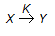# Chemical Engineering - Chemical Reaction Engineering

### Exercise :: Chemical Reaction Engineering - Section 7

26.

In a reversible reaction, a catalyst increases the rate of forward reaction

 A. only. B. to a greater extent than that of the backward reaction. C. and decreases that of the backward reaction. D. and the backward reaction equally.

Explanation:

No answer description available for this question. Let us discuss.

27.

Maximum equilibrium conversion for endothermic reaction is obtained at the __________ temperature.

 A. highest possible B. lowest possible C. intermediate D. room

Explanation:

No answer description available for this question. Let us discuss.

28.

When an exothermic reversible reaction is conducted adiabatically, the rate of reaction

 A. continuously increases. B. continuously decreases. C. passes through a maximum. D. passes through a minimum.

Explanation:

No answer description available for this question. Let us discuss.

29.

For a first order chemical reaction in a porous catalyst, the Thiele modulus is 10. The effectiveness factor is approximately equal to

 A. 1 B. 0.5 C. 0.1 D. 0

Explanation:

No answer description available for this question. Let us discuss.

30.

The rate equation for the reaction represented by,is given by - rx = K1 . Cx/(1 + K2 Cx). At high value of Cx (i.e.., K2Cx > > 1), the order of the reaction and the rate constant are respectively

 A. zero order & K1/K2 B. zero order & K1 C. first order & K1 D. first order & K1/K2